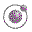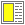Expressions, Types, and Functions - slide 25 : 46

Programs represented as lists
It is a unique property of Lisp that programs are represented as data, using the main data structure of the language: the list
A sample Scheme program from the LAML library:

```(define (as-number x)
(cond ((string? x) (string->number x))
((number? x) x)
((char? x) (char->integer x))
((boolean? x) (if x 1 0))  ; false -> 0, true -> 1
(else
(error
(string-append "Cannot convert to number "
(as-string x))))```
In Scheme it is not intended that the program source should be introspected by the running program

But in other Lisp systems there is easy access to self reflection